# SIGACT Review of: Combinatorics the Rota Way

SIGACT News review of: Combinatorics the Rota Way. Also found on Professor Gasarch’s page and ACM SIGACT News Volume 41, Issue 2 (paywall)

Review of
Combinatorics The Rota Way
by Joseph P.S. Kung, Gian-Carlo Rota and Catherine H. Yan
Cambridge, 2009
Review by
John Mount, jmount@win-vector.com
April 20, 2010

# Introduction

Combinatorics, as it matures, becomes harder to succinctly describe. The field has progressed from the basic study of finite sets and counting techniques to being the discipline where questions involving counting, graphs, connectivity, mappings and partial orders all naturally reside. But the objects that combinatorics studies turn out not to be the correct foundation to support modern combinatorial methods. Many combinatorial methods were dismissed as mere technique until combinatorics expanded to include the natural domains of these methods: lattices, formal power series, valuation rings, matroids and many diverse algebras. One person who pushed hard for this coherence and unity was Gian-Carlo Rota.

An example of a high-school level combinatorial trick is proving the equation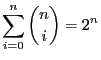by applying the binomial theorem to. This trick is transformed into a method when you recognize that you really should be working in the ring of formal power series and invent the Umbral Calculus. With the Umbral Calculus you can use the equivalence of the following two equations: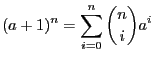(i.e.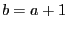is equivalent to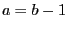) to prove that for any two arbitrary infinite sequences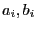the following two statements are also equivalent: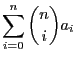for all(1)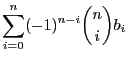for all(2)

For example: we could pickand substitute it into Equation 1. With some work we see this implies.1Then by the Umbral result we know Equation 2 must also be true so we get a new identity: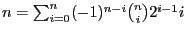. This algebraic production of a new identity is very different than the classical method of “counting two ways” (or being lucky enough to come up with a clever bijection to prove the identity).

# Summary

The book “Combinatorics the Rota Way” is itself hard to succinctly describe. The first and third authors tell of writing this book using notes from the Massachusetts Institute of Technology’s course 18.315 collected over a span of more than 30 years. Gian-Carlo Rota himself was added as a posthumous author. The book itself contains more than a single course-year’s worth of material and is packed very densely.

The book’s emphasis is abstract and algebraic. The exercises are not to teach, but are instead to identify applications of combinatorics in other mathematical disciplines. The book is the product of a strong push to demonstrate many combinatorial methods in their most powerful, but not most obvious, forms. This work is clearly a labor of love and contains some remarkable material. However, due to the large breadth of the work not much time is spent on motivation or on concrete examples.

## Chapter 1: Sets, Functions and Relations

The first chapter covers the definitional foundations of combinatorics: sets, lattices, partial orders, functions and relations. These are the discrete objects that the book will reason about by later building more complicated algebraic objects. This section is very dense and reads like a compressed Bourbaki treatment of discrete mathematics.

One portion of this chapter that is problematic is the section on entropy that seems to serve no purpose other than to prepare the reader for exercise 1.4.10 which demonstrates an abstraction of entropy. Also, exercises 1.2.5(j,k) are needlessly cruel in asking the reader to recreate the Robertson-Seymour graph minor theorem. There have been books where the reader is successfully guided through a major result by exercises, such as the Weak Perfect Graph Theorem in Lovász’s “Combinatorial Problems and Exercises”, but this book is not structured in that manner.

## Chapter 2: Matching Theory

The second chapter is a welcome change in tone and opens with a quote from Harper and Rota describing matching theory and a clever 1979 Putnam exam problem is worked into the exercises and solutions. Central to the chapter is “marriage theorem”, which determines when matchings are possible. Also discussed is Birkhoff’s Theorem, which states that every doubly stochastic matrix is a convex combination of permutations matrices, which relates matchings to matrices. The text is lively and includes a number of well-researched asides, such as the origin of the name “The Hungarian Method.” However, there are some problems with forward reference: for example the reader is asked to work a couple of exercise (2.4.5 and 2.4.6) using the Binet-Cauchy formula, which isn’t discussed at length until chapter 6.

## Chapter 3: Partially Ordered Sets and Lattices

This chapter begins with a very exciting presentation of the Möbius Function (the convolutional inverse of what is essentially the indicator function of a partial order). It is a real pleasure to see this material well presented in a general lattice setting, instead of the more common and specialized number theoretic setting. The chapter moves on to chains (ordered sequences in lattices) and anti-chains (sets of incomparable elements) in partial orders. The authors present Dilworth’s theorem which states that every partial can be covered by a number of chains no larger than the size of the largest anti-chain.2 The chapter continues with Sperner Theory, which relates counting anti-chains to binomial coefficients. Chapter 3 concludes with valuation rings and Möbius Algebras: a transition to the more algebraic style found in Chapter 4.

## Chapter 4: Generating Functions and the Umbral Calculus

This is a key chapter. The book introduces the Umbral Calculus, a transform space automating the manipulation of generating functions. The algebra of delta operators is introduced, which provides an abstraction of differentiation. Finally co-algebras are explored, which abstract the processes of factoring.

A rare (and unfortunate) typo on page-190 mis-defines a basic sequencefor the delta operator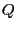as obeying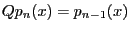instead of the correct equation:. A careful reader can spot the mistake as it is inconsistent with the the subsequent demonstrations and uses.

## Chapter 5: Symmetric Functions and Baxter Algebras

This chapter treats a number of important algebraic topics. Symmetric functions are studied and identified as being the obvious class of functions that contains all of the well know generating functions already studied. Pólya’s Enumeration Theory, which is the method of counting the number of equivalence classes of distinct arrangements, is given a very interesting exposition. But the book skips the classic examples and exercises, such as counting the number of ways to construct distinct necklaces from colored beads, that would be needed for the topic to be fully approachable. Baxter Algebras, which abstract both summation and integration by parts, are introduced and via a study the sequence shift operator. By this point the book has abstract versions of both differentiation and integration, providing a combinatorial groundwork to prove theorems on “the calculus” that are more general than is possible in any one theory of differentiation or integration.

## Chapter 6: Determinants, Matrices and Polynomials

This chapter is most similar to classical polynomial invariant theory, the study of symmetric functions of the roots of polynomials such as the discriminant. A major theme of this chapter is the study of the relations between properties of polynomial coefficients and the locations of roots of the polynomials. The study of matrices brings us to the remarkable Binet-Cauchy Formula for the determinant of a product of matrices. The results are deep, but it is a shame that more time isn’t spent on simple concrete applications such as using the Binet-Cauchy formula to count the number of spanning trees in a graph. This chapter reveals the parts of combinatorics that come from analysis and the study of locations of roots of polynomials (via group theory), in contrast to the parts that come from enumerating finite sets, linear algebra and abstract algebra. This is also the chapter where the exterior algebra, a favorite tool of Rota’s, is most discussed.

A typo on page 275 (a potentially confusing comma in the definition of the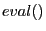operation) can be recovered from because the authors have the nice habit of explicitly calling out the domain and range of functions.

# Opinion

Some important questions about this book are: is Gian-Carlo Rota a coauthor, what is the purpose of the book and who is the best audience?

Gian-Carlo Rota seems appropriately labeled as a co-author, as clearly a lot of his work went into the book. The book is not suitable to be used as an introductory text book or as a reference. It is a book meant to be read. The ideal audience is capable of graduate level mathematics, is comfortable with a high degree of abstraction and algebra and is already familiar with many of the structures and techniques of combinatorics: sets, graphs, matrices, alternating sequences and generating functions. A mathematician or computer scientist wanting to learn more about the science of combinatorics will find a good read here.

The book works best as a second read of the topics covered. If you already know of a combinatorial method, like Pólya’s Enumeration Theory, this book is a good place to find the starting point for an alternate and powerful treatment of the topic. The book admits to not being self contained, and has a few forward-reference problems. However, this is forgivable when you realize the goal of this book is not to teach some easy discrete mathematics before you move on to analysis, but to extract the important combinatorial methods and themes from all of mathematics.

The content is well written, very accurate and well edited. The index is good, but not quite up to the job. The bibliography is very good and divided into three useful sections: papers by Gian-Carlo Rota and coworkers, books for further reading and a section of references.

We close with a extract from the book at hand. Many mathematicians have used the phrase “merely combinatorial proof” as a phrase of dismissal. However, when properly founded, combinatorial proofs are in fact more general than proofs that depend on additional specific details from the original problem domain. The authors take some justifiable pleasure in including points like: “Hilbert’s basis theorem is equivalent to the ‘trivial combinatorial fact’ given in Gordan’s lemma.” This is certainly a taste of combinatorics the Rota way.

#### Footnotes

….1
For this use the binomial theorem to expand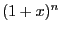, differentiate with respect toand then substitute in.
… anti-chain.2
From this they derive just about the only Ramsey-theoretic style result in the book: any large partial order must have a large chain or large anti-chain.

Disclosure: we did receive a free evaluation copy of the book prior to writing this review.

Categories: Mathematics Opinion

Tagged as:### jmount

Data Scientist and trainer at Win Vector LLC. One of the authors of Practical Data Science with R.